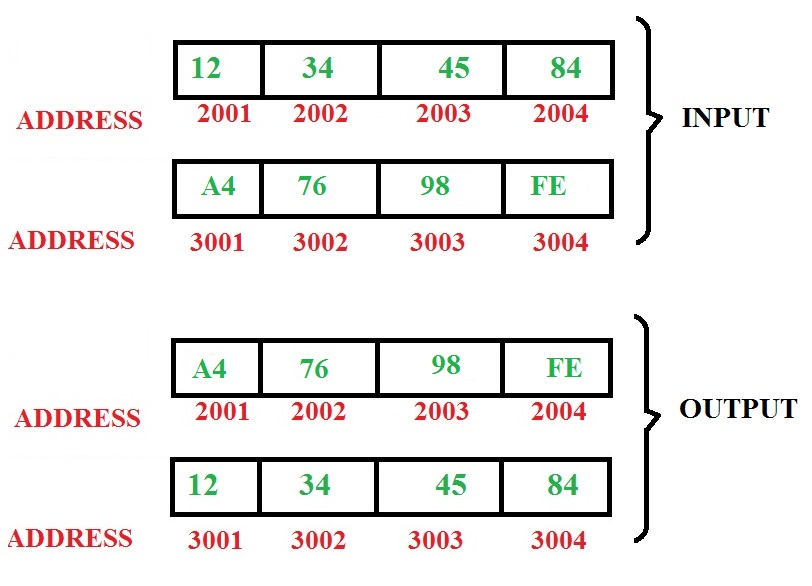# 8085 program to exchange a block of bytes in memory

Problem – Write an assembly level program in 8085 microprocessor to exchange a block of 4 bytes staring from address 2001 with data starting from address 3001.Algorithm –

1. Take a count equal to 4
2. Store the starting address of both blocks in 2 different register pairs
3. Now exchange the contents at the addresses in both register pairs
4. Increment the values of both register pairs
5. Decrements count by 1
6. If count is not equal to 0 repeat steps 3 to 5

2500 LXI D 2001 D <= 20, E <= 01
2503 LXI H 3001 H <= 20, L <= 01
2506 MVI C 04 C <= 04
2508 MOV B, M B <= M[ H-L ]
2509 LDAX D A <= M[ D-E ]
250A MOV M, A M[ H-L ] <= A
250B MOV A, B A <= B
250C STAX D M[ D-E ] <= A
250D INX H [ H-L ] <= [ H-L ] + 1
250E INX D [ D-E ] <= [ D-E ] + 1
250F DCR C C <= C – 1
2510 JNZ 2508 JUMP TO 2508 IF C NOT EQUAL TO 0
2513 HLT STOP THE PROGRAM

Explanation –

1. LXI D 2001 – Loads register pair, that is in this case, D=20 and E=01
LXI H 3001 – H=30 and L=01
2. MVI C 04 – Assigns immediate data, eg.- here C=04
MVI A 45 – assigns A(accumulator) with 45, A=45
3. MOV B, M – Here M is the data in H – L register pair and it serves as an address. Copies content at address stored in M to register B
4. LDAX D – Here Accumulator is loaded with the data stored at address formed by register pair D – E
5. MOV M, A – Here A’s content is copied to address which is stored in M.
MOV A, B – Copies content of register B to A
6. STAX D – Stores the content of A (accumulator) in the address formed by register pair D – E.
7. INX H – Increment the content of register pair H – L
8. INX H – Increment the content of register pair D – E
9. DCR C – Decrements the content of register C
10. JNZ 2508 – If value of register C is not equal to 0 then jump to address 2508
11. HLT – Stop execution of program

GeeksforGeeks has prepared a complete interview preparation course with premium videos, theory, practice problems, TA support and many more features. Please refer Placement 100 for details

My Personal Notes arrow_drop_upCheck out this Author's contributed articles.

If you like GeeksforGeeks and would like to contribute, you can also write an article using contribute.geeksforgeeks.org or mail your article to contribute@geeksforgeeks.org. See your article appearing on the GeeksforGeeks main page and help other Geeks.

Please Improve this article if you find anything incorrect by clicking on the "Improve Article" button below.